2018-07-03 10:15:34 weixin_41783077 阅读数 32611
• ###### matlab 基于阈值分割的区域生长的医学图像分割3讲

matlab 基于阈值分割的区域生长的医学图像分割3讲

186 人正在学习 去看看 刘昱显

（自己写的，需要转载请联系作者，或者标明出处呀，欢迎加微信交流：wx604954）

# 0引言

# 1. 医学图像分割

## 1.1 MR图像

MRI对软组织有很好的成像能力；有非常高的分辨率；具有较高的信噪比；利用不同的脉冲序列可以得到对比度多变的多通道图像，进而用于不同解剖结构的目标分割和分类。然而，在MRI中存在多种伪影，如部分容积、随机场噪声、强度不均匀性、梯度、运动、环绕、吉布斯振铃、磁化性等。此外，相比于CT图像，MRI的获取需要相当长的时间，且通常条件下很难得到统一的图像质量。

## 1.2 CT图像

CT成像中的伪影包括：部分容积效应、条形伪影、运动伪影、束硬化伪影、环状伪影、金属伪影等。由于这些伪影的存在给CT图像分割带来了一定的难度，不同组织部位分割精度也不一样。

## 1.3 医学图像分割的特点

CT和MR图像的分割主要涉及3个相关问题：变化的噪声、像素灰度分类的不确定性及灰度的非均衡性。图像中单一组织的灰度水平一般是逐渐变化的，且其概率密度服从特定的分布函数，该组织对应的图像区域包含有限的像素(或体素)且满足部分容积平均，然而该区域中的单个像素(或体素)的灰度不与任何一类一致，往往被看作混合组织类。

CT和MR图像分割常用的一些方法有：基于阈值、基于区域、基于形变模型、基于模糊及基于神经网络。

# 2. 深度学习

## 2.2 卷积神经网络

CNN运行于局部输入区域的基本操作包括卷积（Convolution）、激活函数（Rectified Linear Units, ReLu）和池化(Pooling),这些操作仅依赖于相关的空间坐标。记 为某层上坐标 的数据向量，下一层中坐标为 的数据向量为 ， 可由以下公式计算：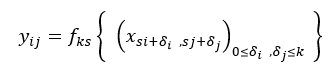(2-2)

（1）卷积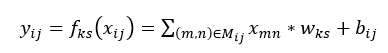(2-3)

（2）激活函数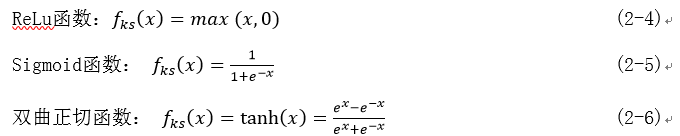（3）池化

## 2.3卷积神经网络在图像分割领域的应用

Wu, Xundong等人提出了基于迭代的卷积神经网络的细胞膜分割方法，通过在细胞膜检测概率图上迭代使用卷积神经网络算法，细胞膜分割精度有了一定的提升。曹贵宝等人提出了用卷积神经网络和随机森林分类器相结合的方法实现了对神经细胞图像的分割，用随机森林分类器代替了传统的softmax分类器，实验中分别训练卷积神经网络模型和分类器模型，并用其学习到的特征训练随机森林分类器，最后完成电子显微图像的神经细胞膜的分割。实验结果的分割准确度明显高于传统的分割算法SVM，而且用随机森林做分类器比用传统的softmax做分类器效果也要好一些。

CERNAZANUGLAVAN等人提出使用卷积神经网络分割X射线图像中的骨骼结构，通过使用四种不同的卷积神经网络提取骨骼X射线图像的图像特征，并将提取到的图像特征用softmax分类器进行分类，从而实现骨骼结构的分割。实验通过对比表明，第四种网络卷积神经网络结构的分割准确率更高。

Su, Hai 等人提出了一种快速扫描卷积神经网络的方法，用于乳腺磁共振图像的分割。与传统卷积神经网络结构不同，该文献在卷积层采用卷积核去卷积整张图像或者上一卷积层提取到的特征图，并重新排列最大池化层，从而减少冗余计算。实验表明，该文献提出的基于快速扫描卷积神经网络的分割方法大大提高的图像分割速度，并保证了分割精度的不变，对乳腺癌实时诊断提出了技术支持。

# 3深度学习在医学图像分割中的应用

## 3.1FCN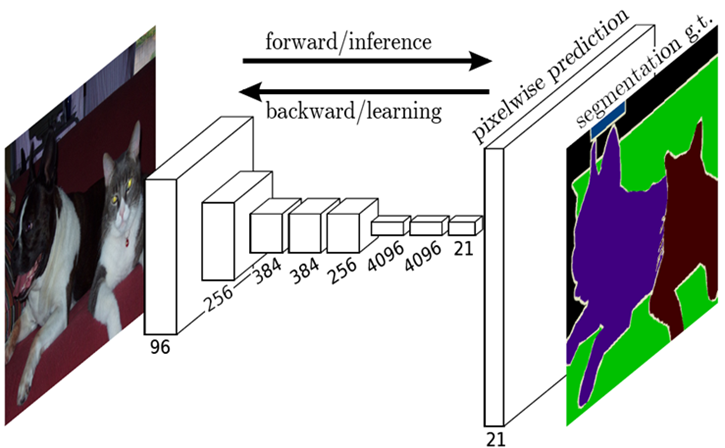FCN在对图像进行分割时，上采样层将特征恢复到原图像的大小，此过程会导致像素定位不精确，从而影响分割结果的准确性。为了解决这一问题，许多研究者采用MRF算法或CRF算法对FCN输出的分割结果进行改进，进一步优化分割结果。

## 3.2U-net

2015年，OlafRonneberger等人提出了U-net网络结构，U-net是基于FCN的一种语义分割网络，适用于做医学图像的分割。

U-net网络结构与FCN网络结构相似，也是分为下采样阶段和上采样阶段，网络结构中只有卷积层和池化层，没有全连接层，网络中较浅的高分辨率层用来解决像素定位的问题，较深的层用来解决像素分类的问题，从而可以实现图像语义级别的分割。与FCN网络不同的是，U-net的上采样阶段与下采样阶段采用了相同数量层次的卷积操作，且使用skip connection结构将下采样层与上采样层相连，使得下采样层提取到的特征可以直接传递到上采样层，这使得U-net网络的像素定位更加准确，分割精度更高。此外，在训练过程中，U-net只需要一次训练，FCN为达到较精确的FCN-8s结构需要三次训练，故U-net网络的训练效率也高于FCN网络。

U-net网络结构如图2所示, 蓝色箭头代表卷积和激活函数, 灰色箭头代表复制剪切操作, 红色箭头代表下采样, 绿色箭头代表反卷积,conv 1X1代表卷积核为1X1的卷积操作。从图中可以看出，U-net网络没有全连接层，只有卷积和下采样。U-net可以对图像进行端到端的分割，即输入是一幅图像, 输出也是一幅图像。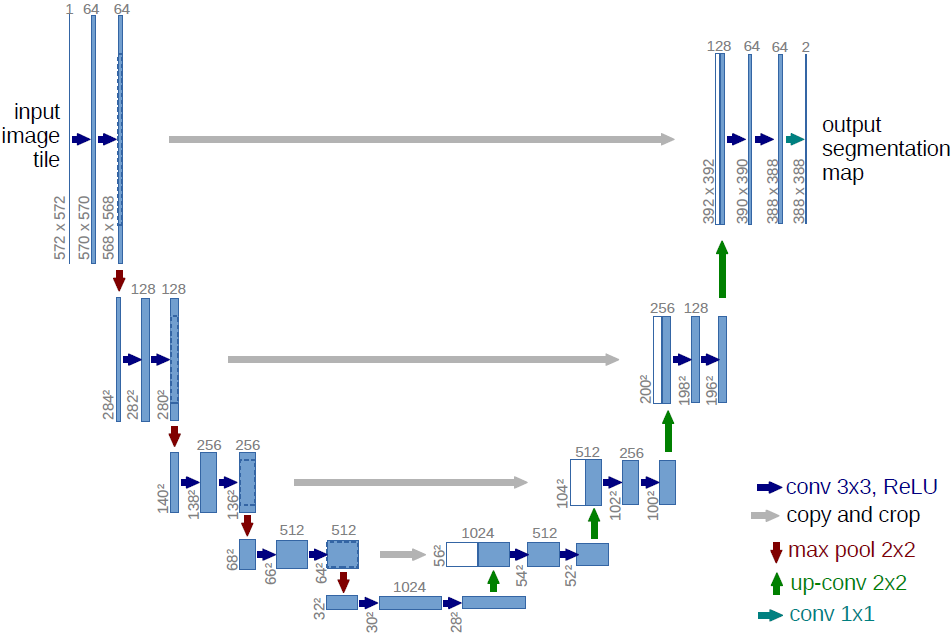U-net网络提出后，在医学图像分割领域表现优秀，许多研究者均采用U-net网络结构做医学图像分割，并在U-net网络结构基础上提出改进。Çiçek等人提出3D U-net网络结构，该结构通过输入3D图像连续的2D切片序列实现3D图像分割。Milletari等人提出了U-net网络结构的一种3D变形结构V-net，V-net结构使用Dice系数损失函数代替传统的交叉熵损失函数，且使用3D卷积核对图像进行卷积，通过1x1x1的卷积核减少通道维数。Drozdzal等人提出在U-net网络结构中不仅可以使用长跳跃连接结构，也可以使用短跳跃连接结构。

# 参考文献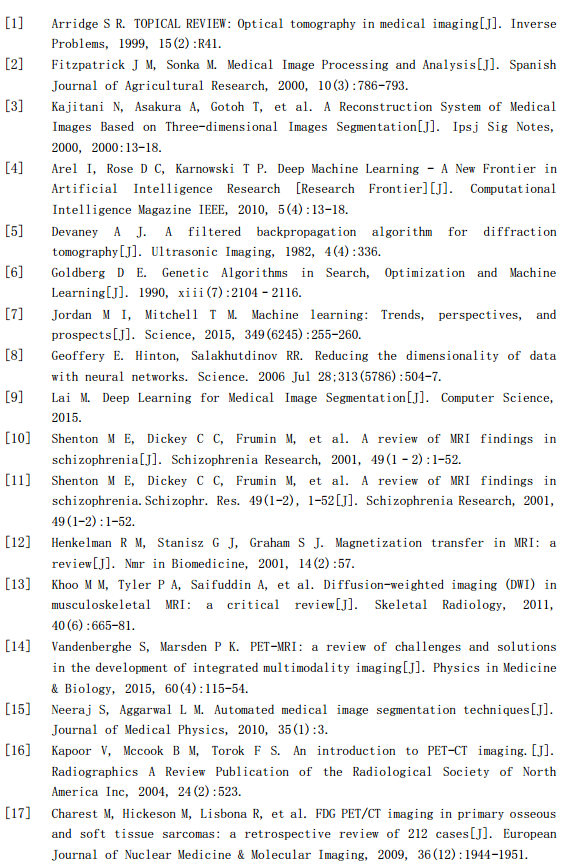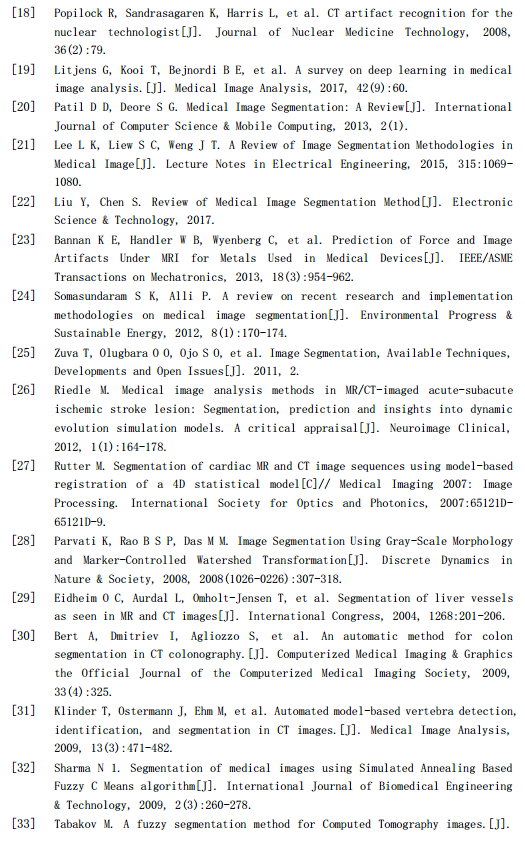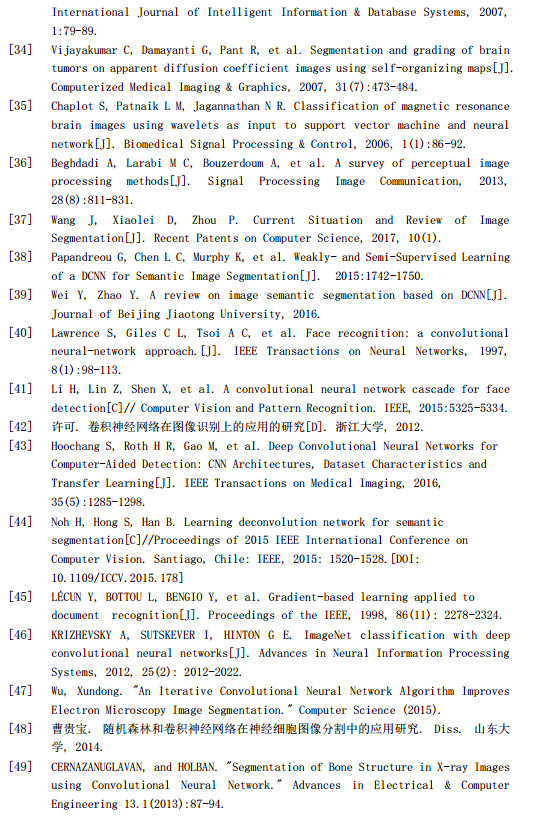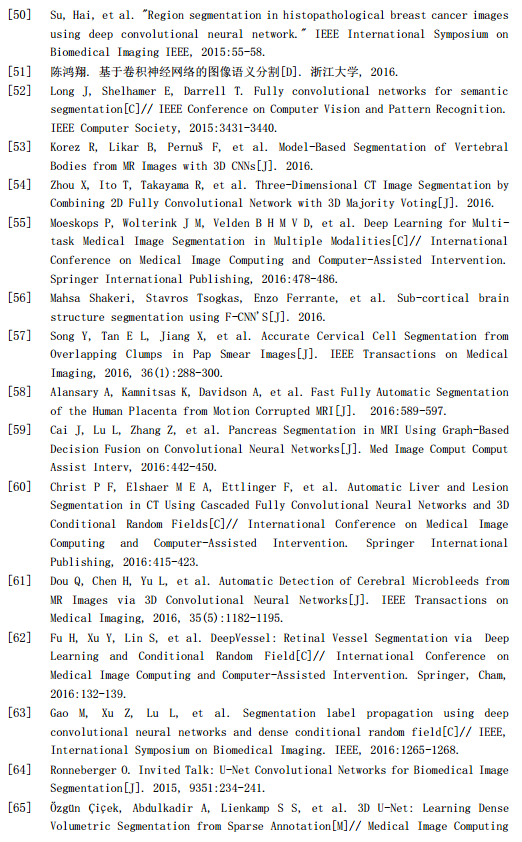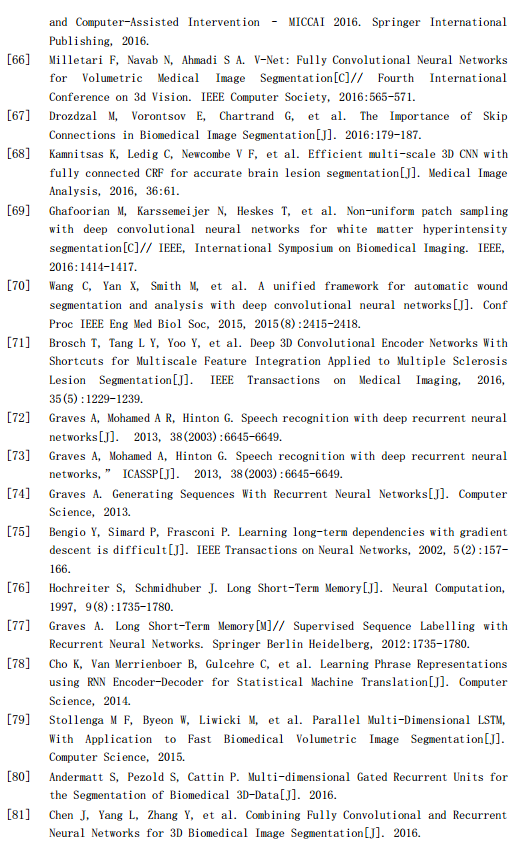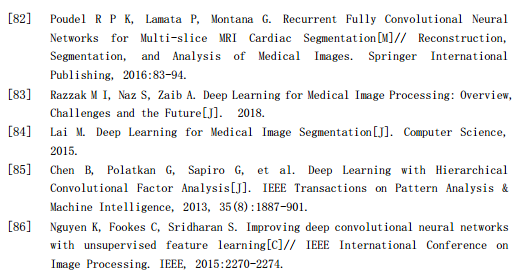2019-09-04 19:36:25 haimianjie2012 阅读数 68
• ###### matlab 基于阈值分割的区域生长的医学图像分割3讲

matlab 基于阈值分割的区域生长的医学图像分割3讲

186 人正在学习 去看看 刘昱显

2019-09-06 17:19:55 OpenSceneGraph 阅读数 163
• ###### matlab 基于阈值分割的区域生长的医学图像分割3讲

matlab 基于阈值分割的区域生长的医学图像分割3讲

186 人正在学习 去看看 刘昱显

1.基于改进的3D-FCN+CRF以及MS-CapsNetGAN实现脑肿瘤图像分割

• FCN-全卷积网络

• MRF-马尔科夫随机场

• CRF-条件随机场

CRF是马尔科夫随机场的特例，它假设马尔科夫随机场中只有和两种变量，一般是给定的，而一般是在给定的条件下我们的输出。这样马尔科夫随机场就特化成了条件随机场。在我们十个词的句子词性标注的例子中，是词，是词性。因此，如果我们假设它是一个马尔科夫随机场，那么它也就是一个CRF。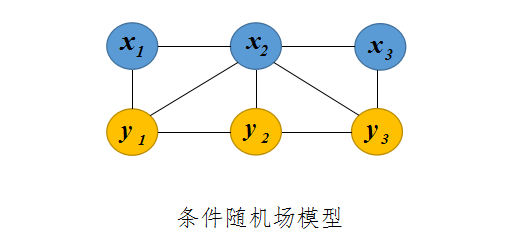①条件随机场保留了隐含马尔可夫模型的一些特性，比如图中的y1,y2,..y1,y2,..等状态的序列还是一个马尔可夫链。
②在图中，顶点x1,y1x1,y1代表一个个随机变量，顶点之间的弧代表他们之间的依赖关系，采用概率分布P(x1,y1)来描述。
③它的特殊性在于变量之间要遵守马尔可夫假设，即每个状态的转移概率只取决于相邻的状态，这一点，它和贝叶斯网络相同。不同之处在于贝叶斯网络是有向图，而条件随机场是无向图，

2.图像分割结果评判标准：

https://blog.csdn.net/woshisunwen/article/details/84308996

3.图像分割方法的分类：基于阈值/边缘/区域/活动轮廓模型/模糊聚类算法/数学形态学/神经网络的图像分割

基于神经网络的图像分割：

CNN 的一个缺点是当卷积特征被馈送到网络的完全连接层时，图像的空间信息丢失。然而，空间信息对于语义分割任务尤其重要。因此，Long 等人提出全卷积网络FCN 克服这个限制。在 FCN 中，CNN 的最终密集连接层由转置卷积层代替，以便将学习的上采样应用于网络内的低分辨率特征映射。该操作可以在执行语义分割的同时恢复输入图像的原始空间维度。类似的网络结构已成功应用于医学成像中的语义分割任务和生物医学图像的分割。从共聚焦显微镜等模式扩展到 3D 生物医学成像数据或磁共振成像。在典型的 FCN 架构中，可以利用跳过连接来连接网络的不同级别，以便保留更接近原始图像的图像特征。这有助于网络实现更详细的分割结果。

4.模糊聚类算法FCM（FCM算法是目前比较流行的一种模糊聚类算法）

• (1)基于模糊关系的分类法：其中包括谱系聚类算法(又称系统聚类法)、基于等价关系的聚类算法、基于相似关系的聚类算法和图论聚类算法等等。它是研究比较早的一种方法，但是由于它不能适用于大数据量的情况，所以在实际中的应用并不广泛。
• (2)基于目标函数的模糊聚类算法：该方法把聚类分析归结成一个带约束的非线性规划问题，通过优化求解获得数据集的最优模糊划分和聚类。该方法设计简单、解决问题的范围广，还可以转化为优化问题而借助经典数学的非线性规划理论求解，并易于计算机实现。因此，随着计算机的应用和发展，基于目标函数的模糊聚类算法成为新的研究热点。
• (3)基于神经网络的模糊聚类算法：它是兴起比较晚的一种算法，主要是采用竞争学习算法来指导网络的聚类过程。

5.体素

6.胶囊网络

PrimaryCaps到DigitCaps层的传播也就是CapsNet和以往CNN操作的最大区别。DigitCaps中一共10个向量，每个向量中元素的个数为16。对这10个向量求模，求得模值最大的那个向量代表的就是图片概率最大的那个分类。因为胶囊网络中：用向量模的大小衡量某个实体出现的概率，模值越大，概率越大。

2019-11-19 22:09:08 weixin_43876801 阅读数 116
• ###### matlab 基于阈值分割的区域生长的医学图像分割3讲

matlab 基于阈值分割的区域生长的医学图像分割3讲

186 人正在学习 去看看 刘昱显

## 导读

随着人工智能，尤其是深度学习技术的迅速发展，基于深度学习的分割方法已在图像分割领域取得了良好的效果，相比传统的机器学习和计算机视觉方法，深度神经网络在分割精度和速度等方面都具有一定的优势。其分割准确度已经超过了传统的分割算法。可以说，深度学习算法在图像处理中具有重要的理论研究意义以及实用价值。然而，目前的深度学习算法主要依靠训练数据，需要大量的手工标记数据用于训练。由于医学图像数据量较大，而且人工标记非常的耗时费力，可能还会有主观意识产生的误差，限制了深度学习方法在医学图像分割领域的应用。
半监督方法通过引入领域知识降低了算法对人工标记数据的需求量，很大程度上缓解了数据标注的难度，是解决数据标记问题的一种重要途径。

## 半监督

半监督学习(Semi-Supervised Learning，SSL)是模式识别和机器学习领域研究的重点问题，是监督学习与无监督学习相结合的一种学习方法。半监督学习使用大量的未标记数据，以及同时使用标记数据，来进行模式识别工作。当使用半监督学习时，将会要求尽量少的人员来从事工作，同时又能够带来比较高的准确性，因此，半监督学习目前正越来越受到人们的重视。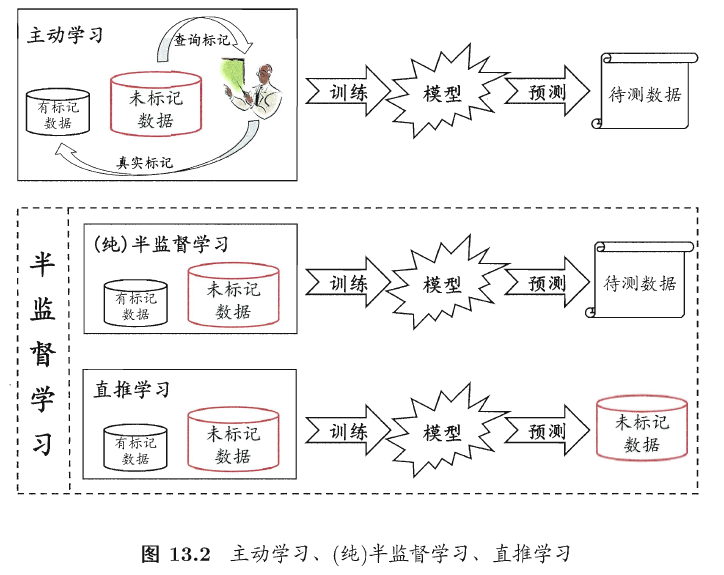详见参考这篇博客：https://www.cnblogs.com/kamekin/p/9683162.html

## 论文1

title：
Transfer Learning from Partial Annotations forWhole Brain Segmentation

## 论文2

title：
Removing segmentation inconsistencies with semi-supervised non-adjacency constraint

下载地址：
https://sciencedirect.xilesou.top/science/article/pii/S1361841519300866

参考：
基于深度学习的医学图像分割技术
百度百科 半监督

医学图像分割深度学习 相关内容

2019-03-24 22:14:55 normol 阅读数 8646
• ###### matlab 基于阈值分割的区域生长的医学图像分割3讲

matlab 基于阈值分割的区域生长的医学图像分割3讲

186 人正在学习 去看看 刘昱显

在之前的一篇博客里：医学图像分割 unet实现(一)，是学习并复现别人的实验。这篇将记录下自己毕设第一阶段的实验。毕设题目为：基于深度学习的肝脏肿瘤分割。
经过几番调整，最终确定：第一阶段分割出腹部图像中的肝脏，作为第二阶段的ROI(region of interest)，第二阶段利用ROI对腹部图像进行裁剪，裁剪后的非ROI区域变成黑色，作为该阶段输入，分割出肝脏中的肿瘤（更新2019-4-2，已做完实验，医学图像分割 基于深度学习的肝脏肿瘤分割 实战(二）。第三阶段用随机场的后处理方法进行优化。
此外，觉得当时的那篇文章写得很不用心，没费多大功夫，复制粘贴为主。我记录的主要原因之一就是觉得这方面入门实战的文章很少，希望让情况类似的同学少走一点弯路。可现在自己写的东西过几天再去看，都会一头雾水。没有从主干思路到细节的分层讲解，没有关键点的介绍。这篇文章开始，将尽我所能，写得利于接受一点。
（不过，自己才开始学习一个多月，python和框架都是临时学的，并不能保证博客里的东西是对的，有错误的话，望指出）

正文：

# 目标

分割出CT腹部图像的肝脏区域。

# 原始数据介绍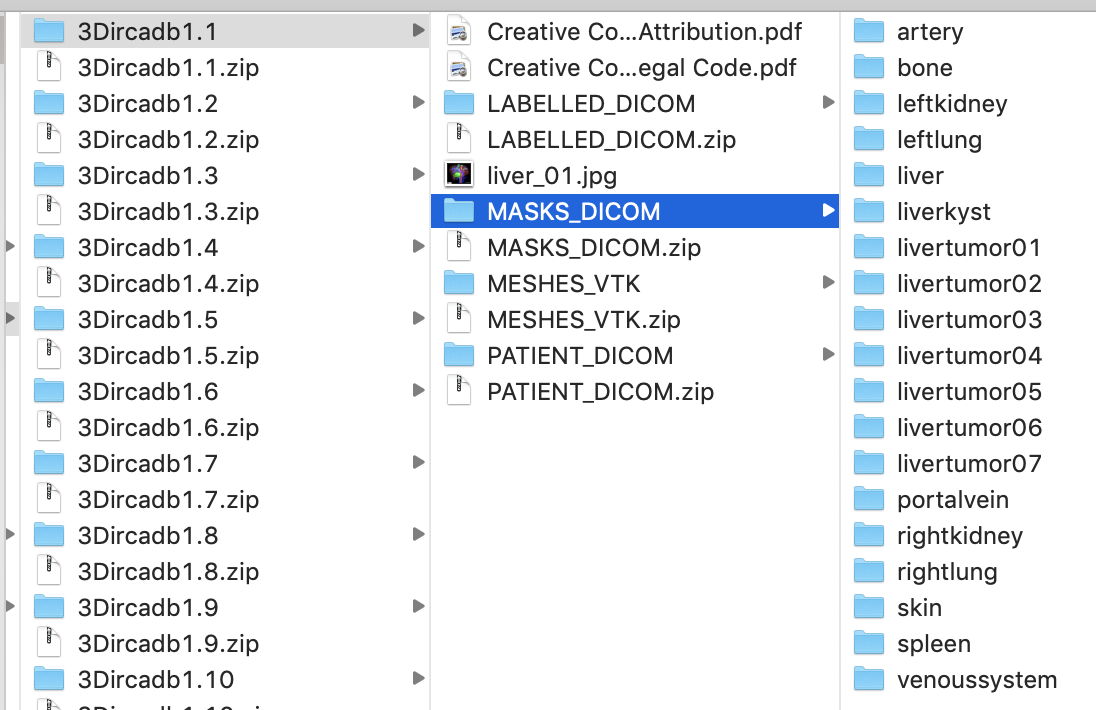PATIENT_DICOM利用软件展示效果如下：一个dcm文件包含129张切片。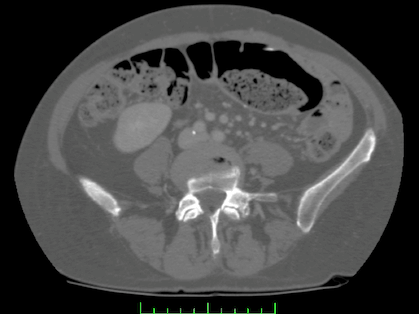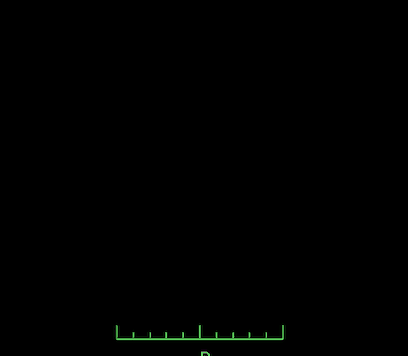# 整体思路

## 1、数据提取

数据读取：
从原始dcm格式读入成我们需要的数组格式

``````#part1
import numpy as np
import pydicom
import os
import matplotlib.pyplot as plt
import cv2
from keras.preprocessing.image import ImageDataGenerator
from HDF5DatasetWriter import HDF5DatasetWriter
from HDF5DatasetGenerator import HDF5DatasetGenerator

for i in range(1,18): # 前17个人作为测试集
full_images = [] # 后面用来存储目标切片的列表
full_livers = [] #功能同上
# 注意不同的系统，文件分割符的区别
liver_slices = [pydicom.dcmread(label_path + '/' + s) for s in os.listdir(label_path)]
# 注意需要排序，即使文件夹中显示的是有序的，读进来后就是随机的了
liver_slices.sort(key = lambda x: int(x.InstanceNumber))
# s.pixel_array 获取dicom格式中的像素值
livers = np.stack([s.pixel_array for s in liver_slices])
image_slices = [pydicom.dcmread(data_path + '/' + s) for s in os.listdir(data_path)]
image_slices.sort(key = lambda x: int(x.InstanceNumber))

""" 省略进行的预处理操作，具体见part2"""

full_images.append(images)
full_livers.append(livers)

full_images = np.vstack(full_images)
full_images = np.expand_dims(full_images,axis=-1)
full_livers = np.vstack(full_livers)
full_livers = np.expand_dims(full_livers,axis=-1)
``````

## 2、数据的预处理

1、将ct值转化为标准的hu值
至于为什么要将值进行转化，这儿就不详细说明，具体可以参考医学图像预处理（三）——windowing(ct对比增强），这篇博文中有一样的`get_pixels_hu`函数
2、窗口化操作
医学图像预处理（三）——windowing(ct对比增强）
3、直方图均衡化

``````def clahe_equalized(imgs,start,end):
assert (len(imgs.shape)==3)  #3D arrays
#create a CLAHE object (Arguments are optional).
clahe = cv2.createCLAHE(clipLimit=2.0, tileGridSize=(8,8))
imgs_equalized = np.empty(imgs.shape)
for i in range(start, end+1):
imgs_equalized[i,:,:] = clahe.apply(np.array(imgs[i,:,:], dtype = np.uint8))
return imgs_equalized
``````

4、归一化
5、仅提取腹部所有切片中包含了肝脏的那些切片，其余的不要
医学图像预处理（四）—— 提取包含目标的切片

``````#part2
# 接part1
images = get_pixels_hu(image_slices)

images = transform_ctdata(images,500,150)

start,end = getRangImageDepth(livers)
images = clahe_equalized(images,start,end)

images /= 255.
# 仅提取腹部所有切片中包含了肝脏的那些切片，其余的不要

total = (end - 4) - (start+4) +1
print("%d person, total slices %d"%(i,total))
# 首和尾目标区域都太小，舍弃
images = images[start+5:end-5]
print("%d person, images.shape:(%d,)"%(i,images.shape))

livers[livers>0] = 1

livers = livers[start+5:end-5]
``````

## 3、数据增强

``````# 可以在part1之前设定好（即循环外）
seed=1
data_gen_args = dict(rotation_range=3,
width_shift_range=0.01,
height_shift_range=0.01,
shear_range=0.01,
zoom_range=0.01,
fill_mode='nearest')

image_datagen = ImageDataGenerator(**data_gen_args)
``````
``````#part3 接part2
image_datagen.fit(full_images, augment=True, seed=seed)
image_generator = image_datagen.flow(full_images,seed=seed)

x=[]
y=[]
i = 0
for x_batch, y_batch in train_generator:
i += 1
x.append(x_batch)
y.append(y_batch)
if i>=2: # 因为我不需要太多的数据
break
x = np.vstack(x)
y = np.vstack(y)
``````

## 4、数据存储

一般而言，数据量较大的话，都会先将原始数据库的东西转化为np或者h5格式的文件，我感觉这样有两个好处，一是真正输入网络训练的时候io量会大大减少(特别是h5很适用于大的数据库）,二是数据分享或者上传至服务器时也方便一点。

实验中会出现两个类，分别是写h5和读h5文件的辅助类
这读文件的类写成了generator，这样可以结合训练网络时，keras的fit_generator来使用，降低内存开销。

``````class HDF5DatasetWriter:
"""用来写数据到h5文件"""
class HDF5DatasetGenerator:
"""用来读h5文件的数据"""
``````

它们具体的实现在python h5文件的读写，因为篇幅问题，所以这儿不详述

h5文件操作需要的包`import h5py`

``````# 可以在part1之前设定好（即循环外）
# 这儿的数量需要提前写好，感觉很不方便，但不知道怎么改，我是先跑了之前的程序，计算了一共有多少
# 张图片后再写的，但这样明显不是好的解决方案
dataset = HDF5DatasetWriter(image_dims=(2782, 512, 512, 1),
outputPath="data_train/train_liver.h5")
``````
``````#part4 接part3
# end of lop
dataset.close()
``````

## 测试数据存储的全部过程

测试数据与上面训练数据的处理过程几乎一样，但测试数据不要进行数据增强

``````full_images2 = []
full_livers2 = []
for i in range(18,21):#后3个人作为测试样本
liver_slices = [pydicom.dcmread(label_path + '/' + s) for s in os.listdir(label_path)]
liver_slices.sort(key = lambda x: int(x.InstanceNumber))
livers = np.stack([s.pixel_array for s in liver_slices])
start,end = getRangImageDepth(livers)
total = (end - 4) - (start+4) +1
print("%d person, total slices %d"%(i,total))

image_slices = [pydicom.dcmread(data_path + '/' + s) for s in os.listdir(data_path)]
image_slices.sort(key = lambda x: int(x.InstanceNumber))

images = get_pixels_hu(image_slices)
images = transform_ctdata(images,500,150)
images = clahe_equalized(images,start,end)
images /= 255.
images = images[start+5:end-5]
print("%d person, images.shape:(%d,)"%(i,images.shape))
livers[livers>0] = 1
livers = livers[start+5:end-5]

full_images2.append(images)
full_livers2.append(livers)

full_images2 = np.vstack(full_images2)
full_images2 = np.expand_dims(full_images2,axis=-1)
full_livers2 = np.vstack(full_livers2)
full_livers2 = np.expand_dims(full_livers2,axis=-1)

dataset = HDF5DatasetWriter(image_dims=(full_images2.shape, full_images2.shape, full_images2.shape, 1),
outputPath="data_train/val_liver.h5")

print("total images in val ",dataset.close())
``````

## 5、构建网络

这一部分不多说，是直接用的别人写好的Unet。
唯一进行的更改，就是Crop的那部分。因为，如果输入图片的高或者宽不能等于2^n(n>=m)，m为网络收缩路径的层数。那么出现的问题就是，下采样的时候进行了取整操作，但是，后续在扩张路径又会有上采样，且上采样的结果会和收缩路径的特征图进行拼接，即long skip connection。若没有达到上面提到的要求，会导致拼接的两个特征图size不同，报错。

``````# partA
import os
import sys
import numpy as np
import random
import math
import tensorflow as tf
from HDF5DatasetGenerator import HDF5DatasetGenerator
from keras.models import Model
from keras.layers import Input, concatenate, Conv2D, MaxPooling2D, Conv2DTranspose,Cropping2D
from keras.callbacks import ModelCheckpoint
from keras import backend as K
from skimage import io

K.set_image_data_format('channels_last')

def dice_coef(y_true, y_pred):
smooth = 1.
y_true_f = K.flatten(y_true)
y_pred_f = K.flatten(y_pred)
intersection = K.sum(y_true_f * y_pred_f)
return (2. * intersection + smooth) / (K.sum(y_true_f) + K.sum(y_pred_f) + smooth)

def dice_coef_loss(y_true, y_pred):
return -dice_coef(y_true, y_pred)

def get_crop_shape(target, refer):
# width, the 3rd dimension
print(target.shape)
print(refer._keras_shape)
cw = (target._keras_shape - refer._keras_shape)
assert (cw >= 0)
if cw % 2 != 0:
cw1, cw2 = int(cw/2), int(cw/2) + 1
else:
cw1, cw2 = int(cw/2), int(cw/2)
# height, the 2nd dimension
ch = (target._keras_shape - refer._keras_shape)
assert (ch >= 0)
if ch % 2 != 0:
ch1, ch2 = int(ch/2), int(ch/2) + 1
else:
ch1, ch2 = int(ch/2), int(ch/2)

return (ch1, ch2), (cw1, cw2)

def get_unet():
inputs = Input((IMG_HEIGHT, IMG_WIDTH , 1))
conv1 = Conv2D(32, (3, 3), activation='relu', padding='same')(inputs)
conv1 = Conv2D(32, (3, 3), activation='relu', padding='same')(conv1)
pool1 = MaxPooling2D(pool_size=(2, 2))(conv1)

conv2 = Conv2D(64, (3, 3), activation='relu', padding='same')(pool1)
conv2 = Conv2D(64, (3, 3), activation='relu', padding='same')(conv2)
pool2 = MaxPooling2D(pool_size=(2, 2))(conv2)

conv3 = Conv2D(128, (3, 3), activation='relu', padding='same')(pool2)
conv3 = Conv2D(128, (3, 3), activation='relu', padding='same')(conv3)
pool3 = MaxPooling2D(pool_size=(2, 2))(conv3)

conv4 = Conv2D(256, (3, 3), activation='relu', padding='same')(pool3)
conv4 = Conv2D(256, (3, 3), activation='relu', padding='same')(conv4)
pool4 = MaxPooling2D(pool_size=(2, 2))(conv4)

conv5 = Conv2D(512, (3, 3), activation='relu', padding='same')(pool4)
conv5 = Conv2D(512, (3, 3), activation='relu', padding='same')(conv5)

up_conv5 = Conv2DTranspose(256, (2, 2), strides=(2, 2), padding='same')(conv5)

ch, cw = get_crop_shape(conv4, up_conv5)

crop_conv4 = Cropping2D(cropping=(ch,cw), data_format="channels_last")(conv4)
up6 = concatenate([up_conv5, crop_conv4], axis=3)
conv6 = Conv2D(256, (3, 3), activation='relu', padding='same')(up6)
conv6 = Conv2D(256, (3, 3), activation='relu', padding='same')(conv6)

up_conv6 = Conv2DTranspose(128, (2, 2), strides=(2, 2), padding='same')(conv6)

ch, cw = get_crop_shape(conv3, up_conv6)
crop_conv3 = Cropping2D(cropping=(ch,cw), data_format="channels_last")(conv3)

up7 = concatenate([up_conv6, crop_conv3], axis=3)
conv7 = Conv2D(128, (3, 3), activation='relu', padding='same')(up7)
conv7 = Conv2D(128, (3, 3), activation='relu', padding='same')(conv7)

up_conv7 = Conv2DTranspose(64, (2, 2), strides=(2, 2), padding='same')(conv7)
ch, cw = get_crop_shape(conv2, up_conv7)
crop_conv2 = Cropping2D(cropping=(ch,cw), data_format="channels_last")(conv2)

up8 = concatenate([up_conv7, crop_conv2], axis=3)
conv8 = Conv2D(64, (3, 3), activation='relu', padding='same')(up8)
conv8 = Conv2D(64, (3, 3), activation='relu', padding='same')(conv8)

up_conv8 = Conv2DTranspose(64, (2, 2), strides=(2, 2), padding='same')(conv8)
ch, cw = get_crop_shape(conv1, up_conv8)
crop_conv1 = Cropping2D(cropping=(ch,cw), data_format="channels_last")(conv1)

up9 = concatenate([up_conv8, crop_conv1], axis=3)
conv9 = Conv2D(32, (3, 3), activation='relu', padding='same')(up9)
conv9 = Conv2D(32, (3, 3), activation='relu', padding='same')(conv9)

conv10 = Conv2D(1, (1, 1), activation='sigmoid')(conv9)

model = Model(inputs=[inputs], outputs=[conv10])

return model
``````

## 6、进行训练并测试

这其中主要包括训练文件与测试文件的读取，Checkpoint回掉函数的设定，fit_generator的使用，模型预测，并对预测结果进行保存。

``````# partB 接partA
IMG_WIDTH = 512
IMG_HEIGHT = 512
IMG_CHANNELS = 1
TOTAL = 2782 # 总共的训练数据
TOTAL_VAL = 152 # 总共的validation数据
# part1部分储存的数据文件
outputPath = './data_train/train_liver.h5' # 训练文件
val_outputPath = './data_train/val_liver.h5'
#checkpoint_path = 'model.ckpt'
BATCH_SIZE = 8 # 根据服务器的GPU显存进行调整

class UnetModel:
def train_and_predict(self):

#

model = get_unet()
model_checkpoint = ModelCheckpoint('weights.h5', monitor='val_loss', save_best_only=True)
# 注：感觉validation的方式写的不对，应该不是这样弄的
model.fit_generator(train_iter,steps_per_epoch=int(TOTAL/BATCH_SIZE),verbose=1,epochs=500,shuffle=True,
#

print('-'*30)
print('-'*30)

print('-'*30)
print('-'*30)

print('-'*30)
print('-'*30)

print('-' * 30)
print('-' * 30)
pred_dir = 'preds'
if not os.path.exists(pred_dir):
os.mkdir(pred_dir)
i = 0

image = (image[:, :, 0] * 255.).astype(np.uint8)
ini = (fixed_test_images[i,:,:,0] *255.).astype(np.uint8)
io.imsave(os.path.join(pred_dir, str(i) + '_ini.png'), ini)
io.imsave(os.path.join(pred_dir, str(i) + '_pred.png'), image)
io.imsave(os.path.join(pred_dir, str(i) + '_gt.png'), gt)
i += 1

unet = UnetModel()
unet.train_and_predict()
``````

模型跑的过程如图。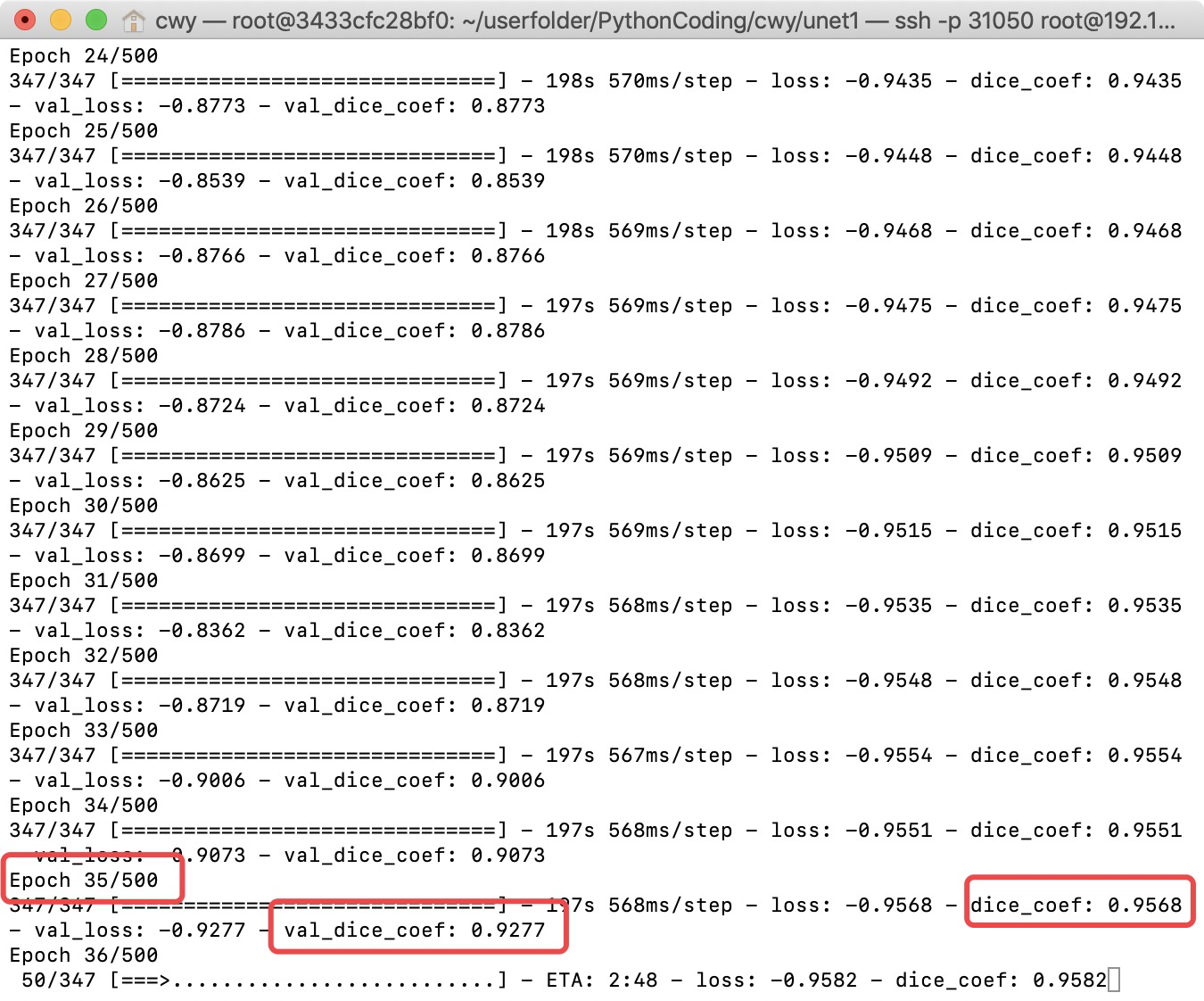预测结果可视化展示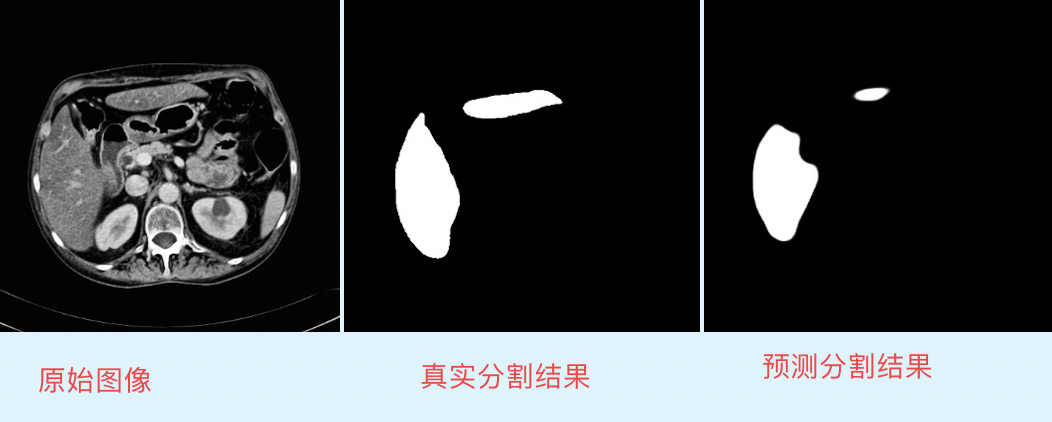医学图像分割深度学习 相关内容

阅读数 4116

博文 来自： weixin_38957591

阅读数 5626

博文 来自： normol

阅读数 940

博文 来自： gefeng1209

阅读数 239

博文 来自： weixin_40779727
没有更多推荐了，返回首页